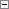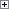Generates a set of random numbers from which the RANDOM Function (Integer) will select a random number.

RANDOMIZE([Seed])

Parameters

Seed

Type: Integer

A number used to create a unique set of numbers.Remarks

If you use the same number as Seed, the same set of numbers is generated. If you omit this optional parameter, RANDOMIZE uses the current system time (total number of milliseconds since midnight). Calling the RANDOMIZE function before the RANDOM function makes the random numbers more unpredictable.

Furthermore, the random generator is specific to each connection so the sequence of numbers that is returned when you call the RANDOM function will be the same after each call to RANDOMIZE with a specific seed.Example

The following example generates random numbers between 1 and 5 by using the Seed from the RANDOMIZE function to initialize the random number generator in the RANDOM function. The RANDOMIZE function uses the data from system clock as the Seed value.

Variable name DataType

x

Integer

Text constant name ENU value

Text000

X=%1Copy Code
RANDOMIZE();
x := RANDOM(5);
MESSAGE(Text000, x);